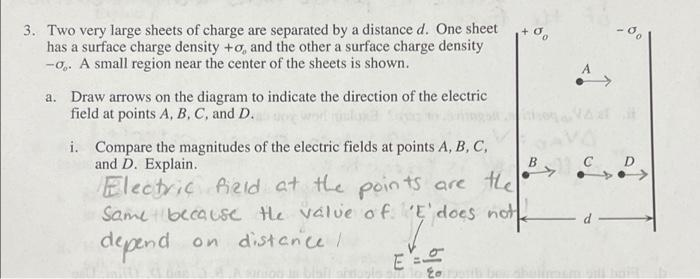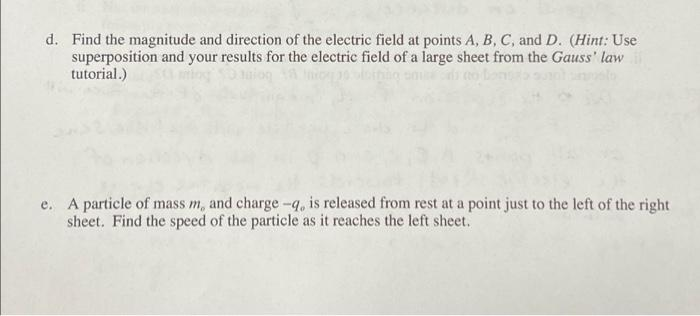# Question Solved1 Answerneed help with number 3 parts d and e please 3. Two very large sheets of charge are separated by a distance d. One sheet has a surface charge density +o, and the other a surface charge density -0.. A small region near the center of the sheets is shown. a. Draw arrows on the diagram to indicate the direction of the electric field at points A, B, C, and D. i. Compare the magnitudes of the electric fields at points A, B, C, and D. Explain. Electric field at the points are the same because the value of E' does not depend on distance B C D E d. Find the magnitude and direction of the electric field at points A, B, C, and D. (Hint: Use superposition and your results for the electric field of a large sheet from the Gauss' law tutorial.) e. A particle of mass m, and charge -9. is released from rest at a point just to the left of the right sheet. Find the speed of the particle as it reaches the left sheet.need help with number 3 parts d and e pleaseTranscribed Image Text: 3. Two very large sheets of charge are separated by a distance d. One sheet has a surface charge density +o, and the other a surface charge density -0.. A small region near the center of the sheets is shown. a. Draw arrows on the diagram to indicate the direction of the electric field at points A, B, C, and D. i. Compare the magnitudes of the electric fields at points A, B, C, and D. Explain. Electric field at the points are the same because the value of E' does not depend on distance B C D E d. Find the magnitude and direction of the electric field at points A, B, C, and D. (Hint: Use superposition and your results for the electric field of a large sheet from the Gauss' law tutorial.) e. A particle of mass m, and charge -9. is released from rest at a point just to the left of the right sheet. Find the speed of the particle as it reaches the left sheet.
More
Transcribed Image Text: 3. Two very large sheets of charge are separated by a distance d. One sheet has a surface charge density +o, and the other a surface charge density -0.. A small region near the center of the sheets is shown. a. Draw arrows on the diagram to indicate the direction of the electric field at points A, B, C, and D. i. Compare the magnitudes of the electric fields at points A, B, C, and D. Explain. Electric field at the points are the same because the value of E' does not depend on distance B C D E d. Find the magnitude and direction of the electric field at points A, B, C, and D. (Hint: Use superposition and your results for the electric field of a large sheet from the Gauss' law tutorial.) e. A particle of mass m, and charge -9. is released from rest at a point just to the left of the right sheet. Find the speed of the particle as it reaches the left sheet.